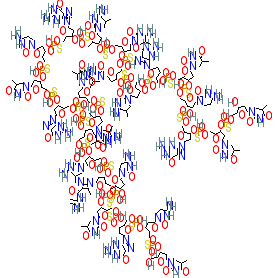#### GtoPdb Ligand ID: 9843

Synonyms: CpG 7909 | CpG7909 | PF 3512676 | PF-3512676 | ProMune | VaxImmune
Compound class: Synthetic organic
Comment: Agatolimod is synthetic CpG oligodeoxynucleotide that acts as a TLR9 agonist . We show the parent molecule, whereas the USAN specifies agatolimod sodium (PubChem CID 56841789), and the synonyms we list may also refer to the sodium congener. Its nucleotide sequence is d(P-thio)(T-C-G-T-C-G-T-T-T-T-G-T-C-G-T-T-T-T-G-T-C-G-T-T).
2D StructurePhysico-chemical PropertiesHydrogen bond acceptors 165 Hydrogen bond donors 55 Rotatable bonds 143 Topological polar surface area 3684.42 Molecular weight 7701.8 XLogP 18.21 No. Lipinski's rules broken 4
SMILES / InChI / InChIKeyCanonical SMILES OCC1OC(CC1OP(=S)(OCC1OC(CC1OP(=S)(OCC1OC(CC1OP(=S)(OCC1OC(CC1OP(=S)(OCC1OC(CC1OP(=S)(OCC1OC(CC1OP(=S)(OCC1OC(CC1OP(=S)(OCC1OC(CC1OP(=S)(OCC1OC(CC1OP(=S)(OCC1OC(CC1OP(=S)(OCC1OC(CC1OP(=S)(OCC1OC(CC1OP(=S)(OCC1OC(CC1OP(=S)(OCC1OC(CC1OP(=S)(OCC1OC(CC1OP(=S)(OCC1OC(CC1OP(=S)(OCC1OC(CC1OP(=S)(OCC1OC(CC1OP(=S)(OCC1OC(CC1OP(=S)(OCC1OC(CC1OP(=S)(OCC1OC(CC1OP(=S)(OCC1OC(CC1OP(=S)(OCC1OC(CC1OP(=S)(OCC1OC(CC1O)n1cc(C)c(=O)[nH]c1=O)O)n1cc(C)c(=O)[nH]c1=O)O)n1cnc2c1nc(N)[nH]c2=O)O)n1ccc(nc1=O)N)O)n1cc(C)c(=O)[nH]c1=O)O)n1cnc2c1nc(N)[nH]c2=O)O)n1cc(C)c(nc1=O)N)O)n1cc(C)c(nc1=O)N)O)n1cc(C)c(nc1=O)N)O)n1cc(C)c(nc1=O)N)O)n1cnc2c1nc(N)[nH]c2=O)O)n1ccc(nc1=O)N)O)n1cc(C)c(=O)[nH]c1=O)O)n1cnc2c1nc(N)[nH]c2=O)O)n1cc(C)c(=O)[nH]c1=O)O)n1cc(C)c(=O)[nH]c1=O)O)n1cc(C)c(nc1=O)N)O)n1cc(C)c(nc1=O)N)O)n1cnc(c1C)C(=O)NC(=C)N)O)n1ccc(nc1=O)N)O)n1cc(C)c(=O)[nH]c1=O)O)n1cnc2c1nc(N)[nH]c2=O)O)n1ccc(nc1=O)N)O)n1cc(C)c(=O)[nH]c1=O Isomeric SMILES OCC1OC(CC1OP(=S)(OCC1OC(CC1OP(=S)(OCC1OC(CC1OP(=S)(OCC1OC(CC1OP(=S)(OCC1OC(CC1OP(=S)(OCC1OC(CC1OP(=S)(OCC1OC(CC1OP(=S)(OCC1OC(CC1OP(=S)(OCC1OC(CC1OP(=S)(OCC1OC(CC1OP(=S)(OCC1OC(CC1OP(=S)(OCC1OC(CC1OP(=S)(OCC1OC(CC1OP(=S)(OCC1OC(CC1OP(=S)(OCC1OC(CC1OP(=S)(OCC1OC(CC1OP(=S)(OCC1OC(CC1OP(=S)(OCC1OC(CC1OP(=S)(OCC1OC(CC1OP(=S)(OCC1OC(CC1OP(=S)(OCC1OC(CC1OP(=S)(OCC1OC(CC1OP(=S)(OCC1OC(CC1OP(=S)(OCC1OC(CC1O)n1cc(C)c(=O)[nH]c1=O)O)n1cc(C)c(=O)[nH]c1=O)O)n1cnc2c1nc(N)[nH]c2=O)O)n1ccc(nc1=O)N)O)n1cc(C)c(=O)[nH]c1=O)O)n1cnc2c1nc(N)[nH]c2=O)O)n1cc(C)c(nc1=O)N)O)n1cc(C)c(nc1=O)N)O)n1cc(C)c(nc1=O)N)O)n1cc(C)c(nc1=O)N)O)n1cnc2c1nc(N)[nH]c2=O)O)n1ccc(nc1=O)N)O)n1cc(C)c(=O)[nH]c1=O)O)n1cnc2c1nc(N)[nH]c2=O)O)n1cc(C)c(=O)[nH]c1=O)O)n1cc(C)c(=O)[nH]c1=O)O)n1cc(C)c(nc1=O)N)O)n1cc(C)c(nc1=O)N)O)n1cnc(c1C)C(=O)NC(=C)N)O)n1ccc(nc1=O)N)O)n1cc(C)c(=O)[nH]c1=O)O)n1cnc2c1nc(N)[nH]c2=O)O)n1ccc(nc1=O)N)O)n1cc(C)c(=O)[nH]c1=O InChI Key GUVMFDICMFQHSZ-UHFFFAOYSA-N
Selectivity at catalytic receptors
Key to terms and symbols Click column headers to sort
Target Sp. Type Action Value Parameter Concentration range (M) Reference
TLR9 Hs Agonist Agonist - - - 3